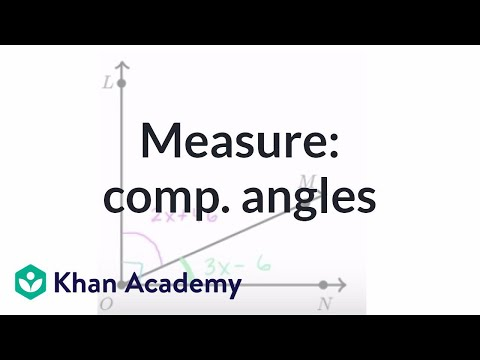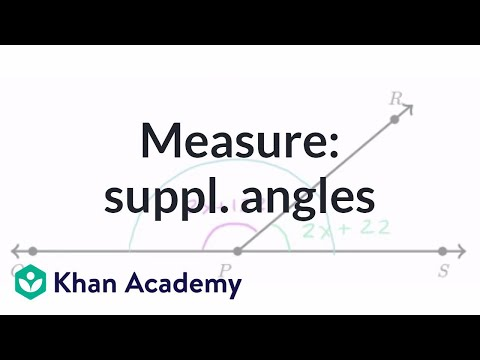Video

# Two-step inequalities (Full video)

Description: Two-step inequalities are slightly more complicated than one-step inequalities. This is a worked example of solving ⅔>-4y-8⅓. Created by Sal Khan and Monterey Institute for Technology and Education. We have the inequality 2/3 is greater than negative 4y minus 8 and 1/3. Now, the next thing I want to do, just because dealing with fractions are a bit of a pain, is multiply both sides of this inequality by some quantity that'll eliminate the fractions. So let's multiply both sides of this equation by 3.

### Other videos you might be interested in### Equation practice with complementary angles (Full video)